OracleAltAir

# Simple Oracle/Jupyter/Keyring/Altair Example¶

In this trivial example we'll be using Jupyter Lab to create this small notebook of Python code. We will see how easy it is to run SQL and analyze those results. The point of the exercise here isn't really the SQL we use but rather how simple it is for developers or analysts who prefer working with code rather than a full blown UI to retrieve and visualise data.

For reference I'm using Python 3.6 and Oracle Database 12c Release 2. You can find the source here

First we'll need to import the required libraries. It's likely that you won't have them installed on your server/workstation. To do that use the following pip command "pip install cx_Oracle keyring pandas altair jupyter jupyter-sql". You should have pip installed and probably be using virtualenv but if you don't I'm afraid that's beyond the scope of this example. Note whilst I'm not importing jupyter and jupyter-sql in this example they are implicitly used.

In :
import cx_Oracle
import keyring
import pandas as pd
import altair as alt


We will be using the magic-sql functionality inside of jupyter-lab. This means we can trivially embed SQL rather than coding the cusrsors and fetches we would typically have to do if we were using straight forward cx_Oracle. This uses the "magic function" syntax" which start with "%" or "%%". First we need to load the support for SQL

In :
%load_ext sql


Next we can connect to the local docker Oracle Database. However one thing we don't want to do with notebooks when working with server application is to disclose our passwords. I use the python module "keyring" which enables you to store the password once in a platform appropriate fashion and then recall it in code. Outside of this notebook I used the keyring command line utility and ran "keyring set local_docker system". It then prompted me for a password which I'm using here. Please be careful with passwords and code notebooks. It's possible to mistakenly serialise a password and then potentially expose it when sharing notebooks with colleagues.

In :
password = keyring.get_password('local_docker','system')


We can then connect to the Oracle Database. In this instance I'm using the cx_Oracle Diriver and telling it to connect to a local docker database running Oracle Database 12c (12.2.0.1). Because I'm using a service I need to specify that in the connect string. I also substitute the password I fetched earlier.

In :
%sql oracle+cx_oracle://system:$password@localhost/?service_name=soe  Out: 'Connected: system@' I'm now connected to the Oracle database as the system user. I'm simply using this user as it has access to a few more tables than a typical user would. Next I can issue a simple select statement to fetch some table metadata. the %%sql result << command retrieves the result set into the variable called result In : %%sql result << select table_name, tablespace_name, num_rows, blocks, avg_row_len, trunc(last_analyzed) from all_tables where num_rows > 0 and tablespace_name is not null   * oracle+cx_oracle://system:***@localhost/?service_name=soe 0 rows affected. Returning data to local variable result  In Python a lot of tabular manipulation of data is performed using the module Pandas. It's an amazing piece of code enabling you to analyze, group, filter, join, pivot columnar data with ease. If you've not used it before I strongly reccomend you take a look. With that in mind we need to take the resultset we retrieved and convert it into a DataFrame (the base Pandas tabular structure) In : result_df = result.DataFrame()  We can see a sample of that result set the the Panada's head function In : result_df.head()  Out: table_name tablespace_name num_rows blocks avg_row_len TRUNC(LAST_ANALYZED) 0 BOOTSTRAP$ SYSTEM 60 3 314 2018-06-14
1 CON$SYSTEM 7068 28 22 2018-06-20 2 OBJ$ SYSTEM 72687 1211 112 2018-06-14
3 TS$SYSTEM 7 7 89 2018-06-14 4 IND$ SYSTEM 2892 1565 89 2018-06-19

All very useful but what if wanted to chart how many tables were owned by each user. To do that we could of course use the charting functionlty of Pandas (and Matplotlib) but I've recently started experimenting with Altair and found it to make much more sense when define what and how to plot data. So lets just plot the count of tables on the X axis and the tablespace name on the Y axis.

In :
alt.Chart(result_df).mark_bar().encode(
x='count()',
y='tablespace_name',
)

Out: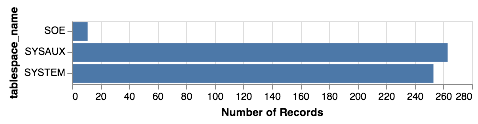Altair makes much more sense to me as a SQL user than some of the cryptic functionality of Matplotlib. But the chart looks really dull. We can trvially tidy it up a few additonal commands

In :
alt.Chart(result_df).mark_bar().encode(
x=alt.Y('count()', title='Number of tables in Tablespace'),
y=alt.Y('tablespace_name', title='Tablespace Name'),
color='tablespace_name'
).properties(width=600, height= 150, title='Tables Overview')

Out: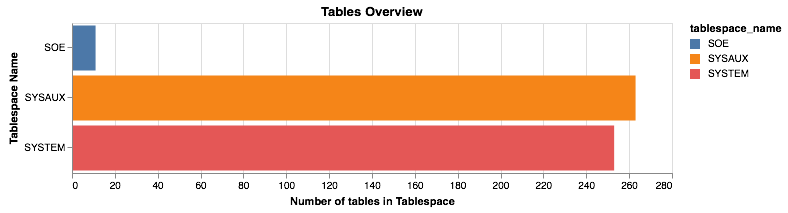Much better but Altair can do significantly more sophisticated charts than a simple bar chart. Next lets take a look at the relationship between the number of rows in a table and the blocks required to store them. It's worth noting that this won't, or at least shouldn't, produce any startling results but it's a useful plot on a near empty database that will give use something to look at. Using another simple scatter chart we get the following

In :
alt.Chart(result_df).mark_point().encode(
y = 'num_rows',
x = 'blocks',
).properties(width=600)

Out: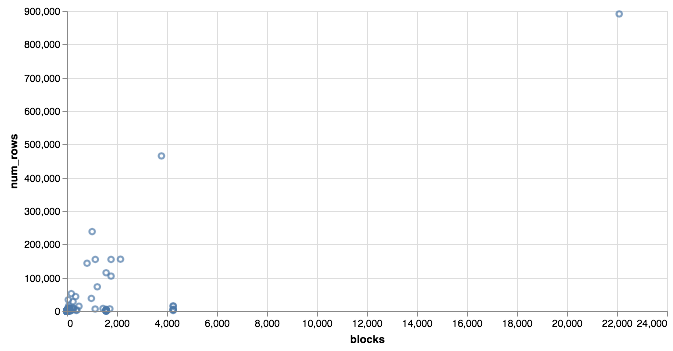Again it's a near empty database and so there's not much going on or at least we have a lot of very small tables and a few big ones. Lets use a logarithmic scale to spread things out a little better and take the oppertunity to brighten it up

In :
alt.Chart(result_df).mark_point().encode(
y = alt.Y('num_rows',scale=alt.Scale(type='log')),
x = alt.X('blocks', scale=alt.Scale(type='log')),
color = 'tablespace_name',
tooltip=['table_name']
).properties(width=600)

Out: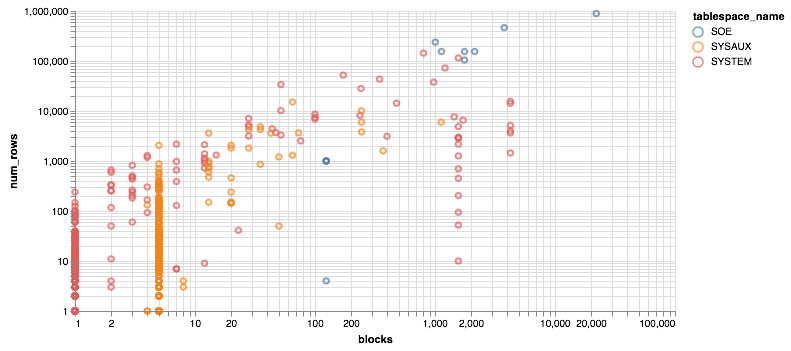Much better... But Altair and the libraries it uses has a another fantastic trick up its sleeve. It can make the charts interactive (zoom and pan) but also enable you to select data in one chart and have it reflected in another. To do that we use a "Selection" object and use this to filter the data in a second chart. Lets do this for the two charts we created. Note you'll need to have run this notebook "live" to see the interactive selection.

In :
interval = alt.selection_interval()

chart1 = alt.Chart(result_df).mark_point().encode(
y = alt.Y('num_rows',scale=alt.Scale(type='log')),
x = alt.X('blocks', scale=alt.Scale(type='log')),
color = 'tablespace_name',
tooltip=['table_name']
).properties(width=600, selection=interval)

chart2 = alt.Chart(result_df).mark_bar().encode(
x='count()',
y='tablespace_name',
color = 'tablespace_name'
).properties(width=600, height= 150).transform_filter(
interval
)

chart1 & chart2

Out: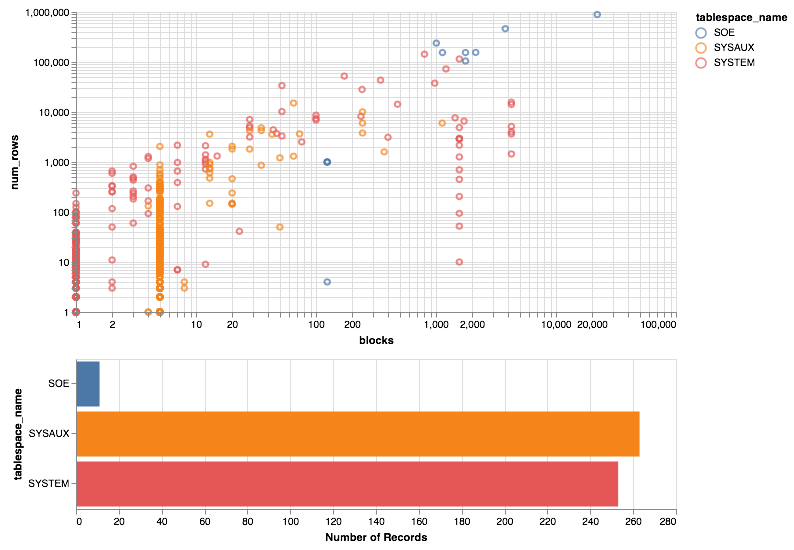And thats it. I'll be using this framework to create a few additional examples in the coming weeks.

The following shows what you would have seen if you had been running the notebook code inside of a browser